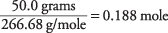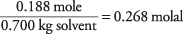## Freezing and Boiling Points

For a solution with a liquid as solvent, the temperature at which it freezes to a solid is slightly lower than the freezing point of the pure solvent. This phenomenon is known as freezing point depression and is related in a simple manner to the concentration of the solute. The lowering of the freezing point is given by

ΔT 1 = K fm

where K f is a constant that depends on the specific solvent and m is the molality of the molecules or ions solute. Table 1 gives data for several common solvents.Use the previous formula and the constant from Table 1 to calculate the temperature at which a solution of 50 grams of sucrose (C 12H 22O 11) in 400 grams of water will freeze. The molecular weight of sucrose is

12(12.01) + 22(1.01) + 11(16.00) = 342.34 g/mole

so, the number of moles of sucrose isand the concentration of the solution in moles per kilogram of water isBy taking the freezing point constant for water as 1.86 from Table

and then substituting the values into the equation for freezing point depression, you obtain the change in freezing temperature:

Δ T f = 1.86°C/m × 0.365 m = 0.68°C

Because the freezing point of pure water is 0°C, the sucrose solution freezes at –0.68°C.

A similar property of solutions is boiling point elevation. A solution boils at a slightly higher temperature than the pure solvent. The change in the boiling point is calculated from

Δ T b = K b m

where K b is the molal boiling point constant and m is the concentration of the solute expressed as molality. The boiling point data for some solvents are provided in Table 1.

Notice that the change in freezing or boiling temperature depends solely on the nature of the solvent, not on the identity of the solute.

One valuable use of these relationships is to determine the molecular mass of various dissolved substances. As an example, perform such a calculation to find the molecular mass of the organic compound santonic acid, which dissolves in benzene or chloroform. A solution of 50 grams of santonic acid in 300 grams of benzene boils at 81.91°C. Referring to Table

for the boiling point of pure benzene, the boiling point elevation is

81.91°C – 80.2°C = 1.71°C = Δ T b

Rearranging the boiling point equation to yield molality and substituting the molal boiling point constant from Table 1, you can derive the molality of the solution:That concentration is the number of moles per kilogram of benzene, but the solution used only 300 grams of the solvent. The moles of santonic acid is found as follows:

0.3 kg × 0.676 mole/kg = 0.203 mole

and the molecular weight is calculated asThe boiling point of a solution was used to determine that santonic acid has a molecular mass of approximately 246. You can also find this value by using the freezing point of the solution.

In the two previous examples, the sucrose and santonic acid existed in solution as molecules, instead of dissociating to ions. The latter case requires the total molality of all ionic species. Calculate the total ionic molality of a solution of 50.0 grams of aluminum bromide (AlBr 3) in 700 grams of water. Because the gram formula weight of AlBr 3 is

26.98 + 3(79.90) = 266.68 g/mole

the amount of AlBr 3 in the solution isThe concentration of the solution with respect to AlBr 3 formula units isEach formula unit of the salt, however, yields one Al 3+ and three Br ions:

AlBr 3 ( s) → Al 3+ ( aq) + 3Br ( aq)

So, the concentrations of the ions are

Al 3+ = 0.268 molal

Br = 3(0.268) = 0.804 molal

Al 3+ + Br = 1.072 molal

The total concentration of ions is four times that of the salt. When calculating the change in freezing point or boiling point, the concentration of all the solute particles must be used, whether they are molecules or ions. The concentration of the ions in this solution of AlBr 3 is 1.072 molal, and this molality would be used to calculate Δ T f and Δ T b.

• Calculate the boiling point of a solution of 10 grams of sodium chloride in 200 grams of water.
• A solution of 100 grams of brucine in 1 kg chloroform freezes at –64.69°C. What is the molecular weight of brucine?